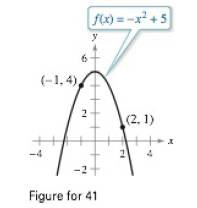Chapter 4.2, Problem 41ECalculus: Early Transcendental Fun...

7th Edition
Ron Larson + 1 other
ISBN: 9781337552516

Solutions

Chapter
SectionCalculus: Early Transcendental Fun...

7th Edition
Ron Larson + 1 other
ISBN: 9781337552516
Textbook Problem

Mean Value Theorem Consider the graph of the function f ( x ) = − x 2 + 5 (see figure).(a) Find the equation of the secant line joining the points ( − 1 , 4 ) and ( 2 , 1 ) .(b) Use the Mean Value Theorem to determine a point c in the interval ( − 1 , 2 ) such that the tangent line at c is parallel to the secant line.(c) Find the equation of the tangent line through c.(d) Use a graphing utility to graph f. the secant line, and the tangent line.(a)

To determine

To calculate: The equation of the secant line joining the points (-1, 4) and (2, 1) considering the given graph of the function f(x)=x2+5.

Explanation

Given: f(x)=x2+5

Refer the image in the given question.

Formula used:

yy1=m(xx1)

Calculation:

The equation of the line connecting the points is,

(b)

To determine
The point c such that the tangent line at c is parallel to the secant line by considering the given graph of the function f(x)=x2+5.

(c)

To determine

To calculate: The equation of tangent line by considering the given graph of the function f(x)=x2+5.

(d)

To determine

To graph: The function f(x)=x2+5 with tangent and secant lines.

Still sussing out bartleby?

Check out a sample textbook solution.

See a sample solution

The Solution to Your Study Problems

Bartleby provides explanations to thousands of textbook problems written by our experts, many with advanced degrees!

Get Started